# Math

Library of Real mathematical functions as input/output blocks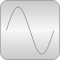# Package Contents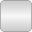UnitConversions Conversion blocks to convert between SI and non-SI unit signals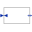InverseBlockConstraints Construct inverse model by requiring that two inputs and two outputs are identical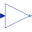Gain Output the product of a gain value with the input signal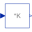MatrixGain Output the product of a gain matrix with the input signal vector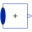MultiSum Sum of Reals: y = k*u + k*u + ... + k[n]*u[n]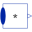MultiProduct Product of Reals: y = u*u* ... *u[n]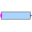MultiSwitch Set Real expression that is associated with the first active input signal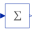Sum Output the sum of the elements of the input vector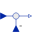Feedback Output difference between commanded and feedback input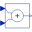Add Output the sum of the two inputs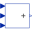Add3 Output the sum of the three inputs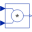Product Output product of the two inputs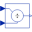Division Output first input divided by second input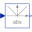Abs Output the absolute value of the input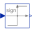Sign Output the sign of the input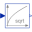Sqrt Output the square root of the input (input >= 0 required)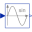Sin Output the sine of the input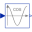Cos Output the cosine of the input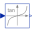Tan Output the tangent of the input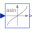Asin Output the arc sine of the input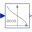Acos Output the arc cosine of the input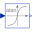Atan Output the arc tangent of the input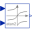Atan2 Output atan(u1/u2) of the inputs u1 and u2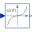Sinh Output the hyperbolic sine of the input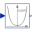Cosh Output the hyperbolic cosine of the input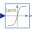Tanh Output the hyperbolic tangent of the input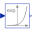Exp Output the exponential (base e) of the input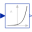Power Output the power to a base of the input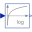Log Output the logarithm (default base e) of the input (input > 0 required)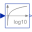Log10 Output the base 10 logarithm of the input (input > 0 required)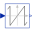WrapAngle Wrap angle to interval ]-pi,pi] or [0,2*pi[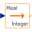RealToInteger Convert Real to Integer signal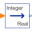IntegerToReal Convert Integer to Real signals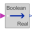BooleanToReal Convert Boolean to Real signal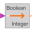BooleanToInteger Convert Boolean to Integer signal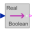RealToBoolean Convert Real to Boolean signal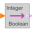IntegerToBoolean Convert Integer to Boolean signal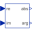RectangularToPolar Convert rectangular coordinates to polar coordinates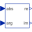PolarToRectangular Convert polar coordinates to rectangular coordinates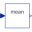Mean Calculate mean over period 1/f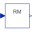RectifiedMean Calculate rectified mean over period 1/f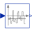ContinuousMean Calculates the empirical expectation (mean) value of its input signal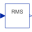RootMeanSquare Calculate root mean square over period 1/f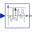Variance Calculates the empirical variance of its input signal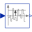StandardDeviation Calculates the empirical standard deviation of its input signal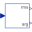Harmonic Calculate harmonic over period 1/f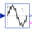TotalHarmonicDistortion Output the total harmonic distortion (THD)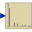RealFFT Sampling and FFT of input u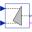Pythagoras Determines the hypotenuse or leg of a right triangle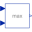Max Pass through the largest signal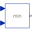Min Pass through the smallest signal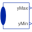MinMax Output the minimum and the maximum element of the input vector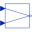LinearDependency Output a linear combination of the two inputs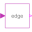Edge Indicates rising edge of Boolean signal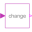BooleanChange Indicates Boolean signal changing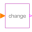IntegerChange Indicates integer signal changing

# Information

This information is part of the Modelica Standard Library maintained by the Modelica Association.

This package contains basic mathematical operations, such as summation and multiplication, and basic mathematical functions, such as sqrt and sin, as input/output blocks. All blocks of this library can be either connected with continuous blocks or with sampled-data blocks.